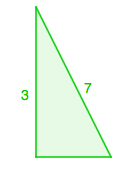### Home > PC > Chapter 11 > Lesson 11.2.2 > Problem11-91

11-91.

If $\sin x = \frac { 3 } { 7 }$ and $x \in [ \frac { \pi } { 2 } , \pi ]$, find the exact values of $\cos x$, $\tan x$, $\cot x$, and $\sin 2x$.

Draw a diagram.Find the length of the missing side.

Then find the exact values of the other trigonometric functions.
Make sure to pay attention to the quadrant.